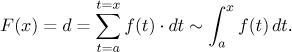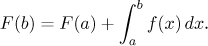Here we will use a physics setting to see why the fundamental theorems of calculus could be true.

Let f (t) be a function defined on [a,b]. We will use the following interpretation:

Imagine that you are driving along a highway and at time t = a, you pass a milepost that says "000". You drive on and record the instantaneous speed on your speedometer as f (t), until time t = b. What is your position F(x) at time t = x along the highway, as measured by mileposts? For details on this setup, see this note in Introduction to functions, but note that to illustrate the Fundamental theorem properly we had to relabel functions. The position that we call F here is called r in that note.

When you drive for t hours at a constant speed v, you cover d = vt miles. However, this cannot be used in our case, since the velocity changes all the time. Since this is a real-life situation, we may assume that f is a "nice" function. This means that if, at some time t, you look at it over some really tiny time interval dt, the velocity almost does not change. Therefore, the change of your position ds during the time segment dt is f (t) times dt. The total change in position (displacement) is obtained by summing all the tiny changes over all possible time segments dt between t = a and t = x:Now we also see why we cannot use x as a variable in the integral when we use it as a limit of this integral. When we fix one such x, we still want to be able to move along time as we add up the small velocity contributions, therefore we need another variable.

In any case, we just saw that the position F(x) is given by the above integral. Since we know from physics that the derivative of displacement gives instantaneous velocity, we get F ′ = f and F is an antiderivative of f, exactly as claimed by TFC 1.

Now imagine that at time t = a, the milepost does not say "000" but some other number. We will again use F to record our position along the highway based on mileposts, in particular, F(a) shows our position at the beginning. From physics we know that F is an antiderivative of the velocity f. The displacement (distance we drove) between times t = a and t = b can be calculated using the position function F, but also summing up the velocities as above, and we getThis is exactly the Newton-Leibniz formula.

This equality is sometimes stated in this way:In our interpretation it means the following: To find your position at time b, you start with the position at time a and add all the displacements corresponding to your instantaneous velocity between times a and b.